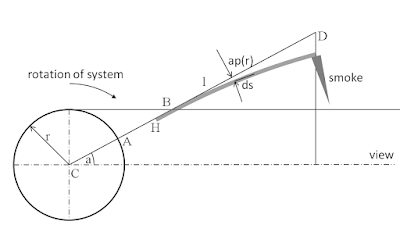## Calculation of Dip 792d with Star lifting

This post is the second part of the calculations to understand the shape of the dip at day 792 of Boyajian's star (KIC 8462852). For part one visit post: "Do we see Star lifting".

### The Beam is Bent

The Dip day 792 is not symmetrical, therefore we have to introduce a beam, that is not perfect on a straight line from the center of the star. it is not difficult, to find reasons why the beam of the matter is bent in a direction. A simple reason is in Kepler dynamics during departure from the surface.
As long as we don't understand the process in detail, I will again start with a simple model and try to fit the model with the data.
Figure 1 describes the new model of the beam. The beam starts at the surface of the star, and with height H it starts to have an optical density due to reduced temperature and therefore is no longer ionized.Fig 1: Properties of the physical model.

The beam is now modeled with small fractions of the length ds which are distorted against the direction angle depending on the distance l. At the end of the beam, the "smoke" comes to a rest and is distributed in this orbit by an exponential law, the density is maximal at the beam end and decays with distance in the bending direction.
For the simulation, the beam contains 105 elements. Each represents a beam length of 5 star radii, The first 5 star radii (first element in the simulation) are transparent due to the high temperature near the star surface.
Each simulated beam element has a transparency of 0,9984722. Each element is a little bit bent by
ap = -0,3971 day.
If an element is within the line of sight between Kepler and KIC 8462852, the resulting transparency is calculated by the multiplication of the transparency of every element.
At the end of the beam, the material enters an orbit, for some reason, and is accumulated. The optical density of the accumulated material decays by an exponential function d = d0 exp(a*w) thereby d0 = 0,001588, w = 3,30025 [1/day]. (The unit hour is used and could be converted in an angle if the distance and rotation period would be known). The value of the simulation was always averaged over 2.5h to adopt a little bit the shape of the unknown optical elements.
The result of this simple physical simulation is shown in fig 2:Fig 2: Measured and simulated flux using the model
The model, containing only simple and plausible parameters fits within the limits of the Kepler data precision. The value of the free parameters, namely bending, optical density and the exponential function, were optimized manually.

I did not expect a result with such a low error.

There are some aspects not included in the simulation: an exact cross-section of the beam, surface flux of the star (it was assumed to be constant).

### Other posts related to Tabbys Star

Dips part two
A simple model of Dip 792